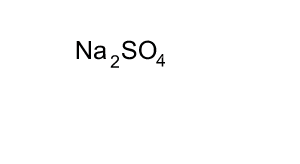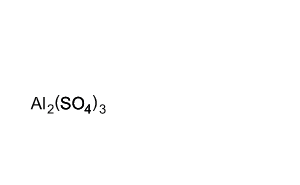Chemical formulae

Chemical formulae describe the composition of compounds and elements. For example, sulfuric acid has the chemical formula H2SO4. Looking at the formula we can tell two things about sulfuric acid.

- It is formed from three different elements, namely, sulfur(S), oxygen(O) and hydrogen(H).

- Each unit of sulfuric acid consists of one atom of sulfur, four atoms of oxygen and two atoms of hydrogen.

Consider the compound sodium sulfate (Na2SO4)

Looking at the formula we can tell that it is made up of sodium, sulfur and oxygen. Every unit of sodium sulfate has two atoms of sodium, one atom of sulfur and four atoms of oxygen.Consider the compound aluminium sulfate. Its formula is Al2(SO4)3. What is the signisficance of the brackets. The brackets tell us that there are more than one SO4 units. In this case we have three units of SO4.
So, from the formula we can see that:

- the compound is made up of aluminium, sulfur and oxygen.

- each unit of aluminium sulfate is composed of two atoms of aluminium, three atoms of sulfur and twelve atoms of oxygen.When writing formulae we use the chemical symbols for each element. It is best to try and remember the first twenty elements and there symbols.

 Name of element Symbol Hydrogen H Helium He Lithium Li Berylium Be Boron B Carbon C Nitrogen N Oxygen O Fluorine F Neon Ne Sodium Na Magnesium Mg Aluminium Al Silicon Si Phosphorus P Sulfur S Chlorine Cl Argon Ar Potassium K Calcium Ca
Fill in the table below
 Compound Element present Number of atoms of each element Ca(NO3)2 Hydrogen Lithium Sodium Nitrogen Oxygen Fluorine Sulfur Chlorine Calcium Aluminium Hydrogen Lithium Sodium Nitrogen Oxygen Fluorine Sulfur Chlorine Calcium Aluminium Hydrogen Lithium Sodium Nitrogen Oxygen Fluorine Sulfur Chlorine Calcium Aluminium 1 2 3 4 5 6 7 8 9 10 11 12 1 2 3 4 5 6 7 8 9 10 11 12 1 2 3 4 5 6 7 8 9 10 11 12 K2SO4 Hydrogen Lithium Sodium Nitrogen Oxygen Fluorine Sulfur Chlorine Calcium Aluminium Potassium Hydrogen Lithium Sodium Nitrogen Oxygen Fluorine Sulfur Chlorine Calcium Aluminium Potassium Hydrogen Lithium Sodium Nitrogen Oxygen Fluorine Sulfur Chlorine Calcium Aluminium Potassium 1 2 3 4 5 6 7 8 9 10 11 12 1 2 3 4 5 6 7 8 9 10 11 12 1 2 3 4 5 6 7 8 9 10 11 12 NH4NO3 Hydrogen Lithium Sodium Nitrogen Oxygen Fluorine Sulfur Chlorine Calcium Aluminium Hydrogen Lithium Sodium Nitrogen Oxygen Fluorine Sulfur Chlorine Calcium Aluminium Hydrogen Lithium Sodium Nitrogen Oxygen Fluorine Sulfur Chlorine Calcium Aluminium 1 2 3 4 5 6 7 8 9 10 11 12 1 2 3 4 5 6 7 8 9 10 11 12 1 2 3 4 5 6 7 8 9 10 11 12
What is the formula of this molecule?Continue